# Light – reflection refraction

31 de Dec de 2021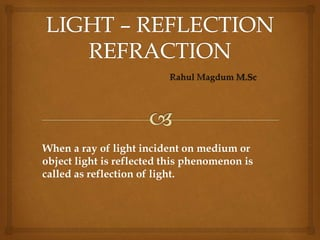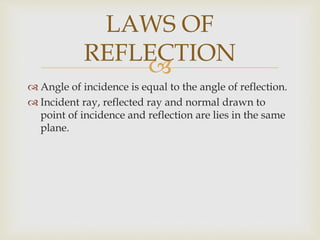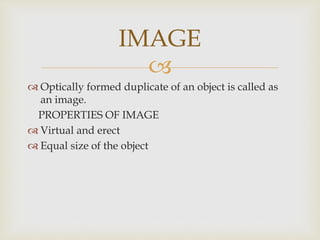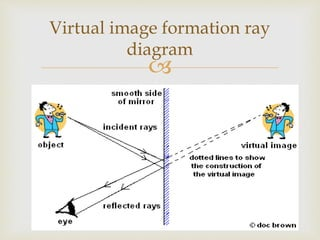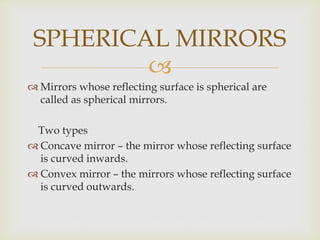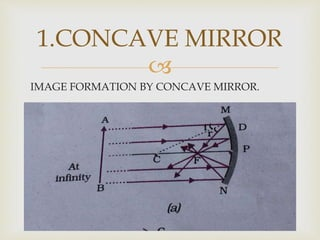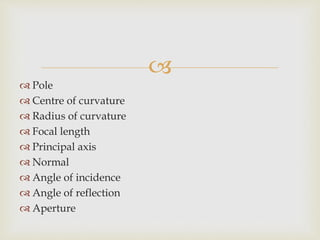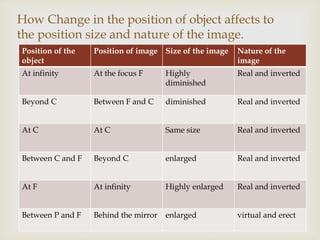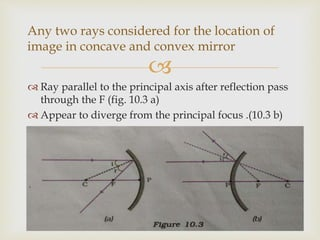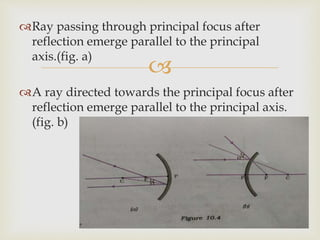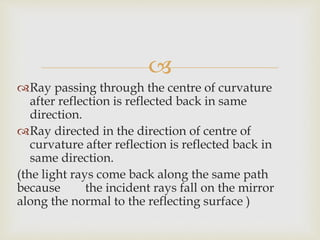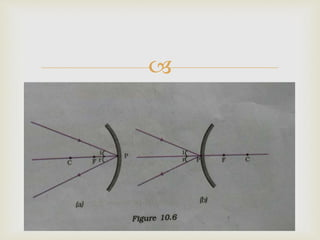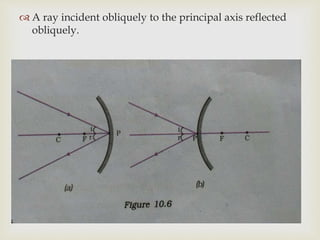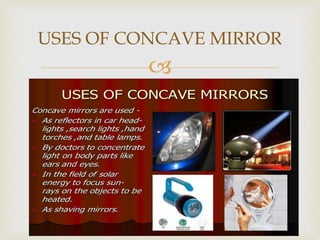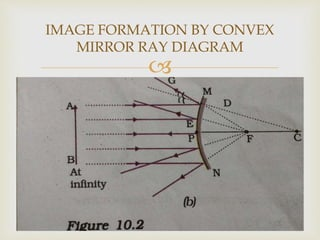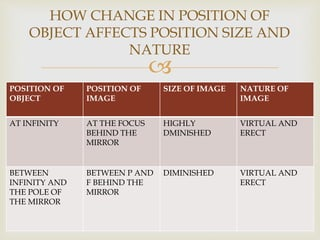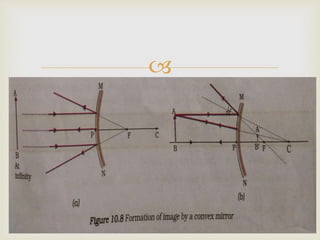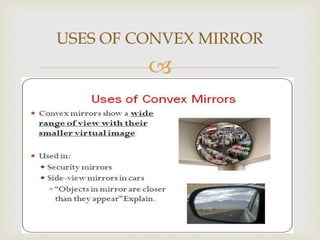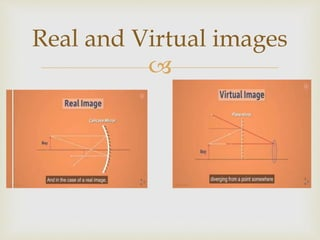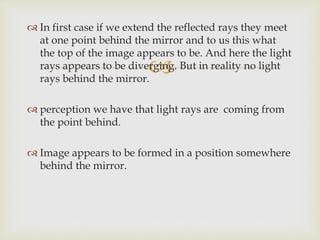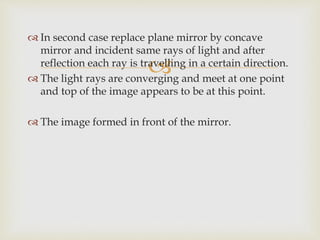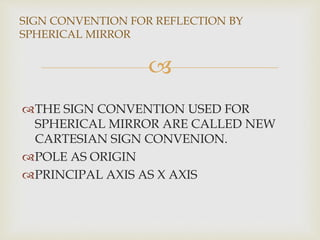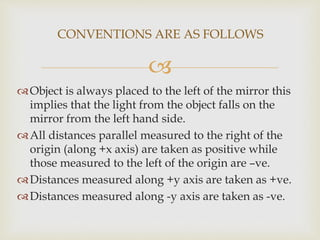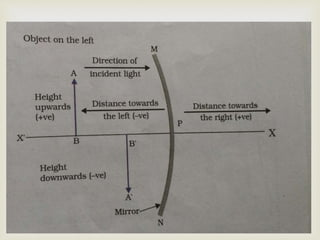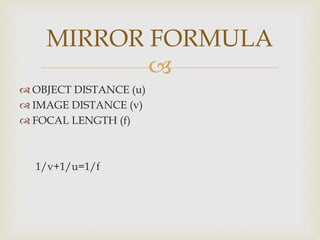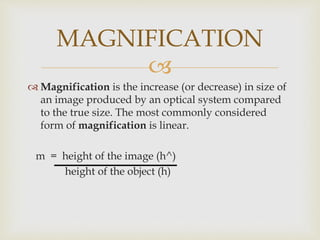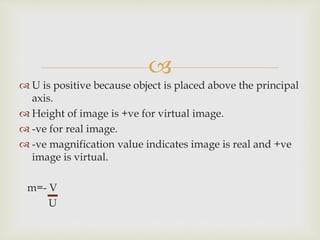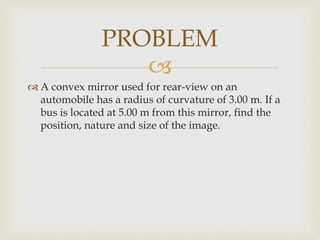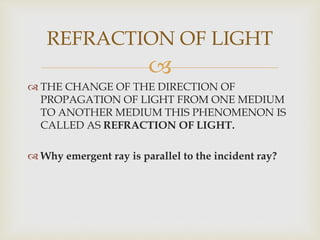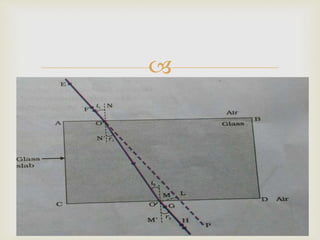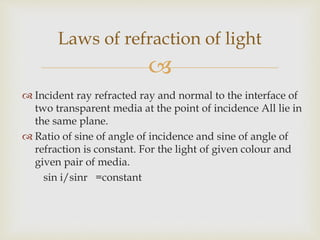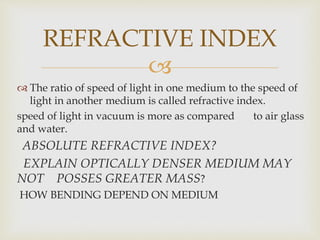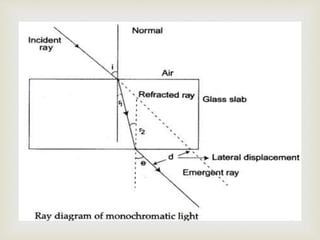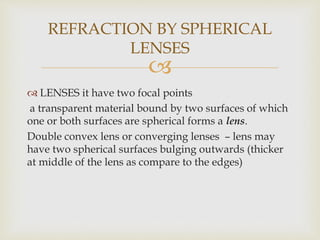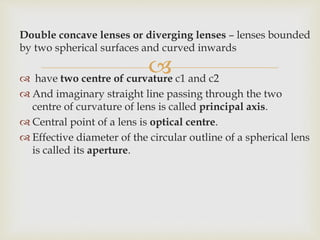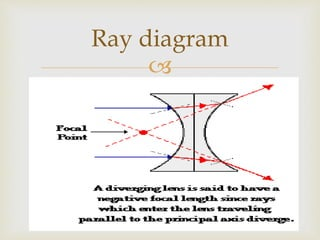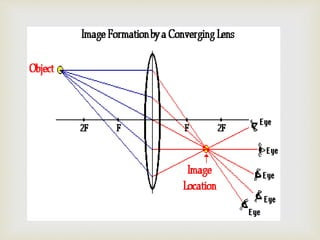1 de 37

### Light – reflection refraction

• 1. When a ray of light incident on medium or object light is reflected this phenomenon is called as reflection of light.
• 2.   Angle of incidence is equal to the angle of reflection.  Incident ray, reflected ray and normal drawn to point of incidence and reflection are lies in the same plane. LAWS OF REFLECTION
• 3.   Optically formed duplicate of an object is called as an image. PROPERTIES OF IMAGE  Virtual and erect  Equal size of the object IMAGE
• 4.  Virtual image formation ray diagram
• 5.   Mirrors whose reflecting surface is spherical are called as spherical mirrors. Two types  Concave mirror – the mirror whose reflecting surface is curved inwards.  Convex mirror – the mirrors whose reflecting surface is curved outwards. SPHERICAL MIRRORS
• 6.  IMAGE FORMATION BY CONCAVE MIRROR. 1.CONCAVE MIRROR
• 7.   Pole  Centre of curvature  Radius of curvature  Focal length  Principal axis  Normal  Angle of incidence  Angle of reflection  Aperture
• 8.  Position of the object Position of image Size of the image Nature of the image At infinity At the focus F Highly diminished Real and inverted Beyond C Between F and C diminished Real and inverted At C At C Same size Real and inverted Between C and F Beyond C enlarged Real and inverted At F At infinity Highly enlarged Real and inverted Between P and F Behind the mirror enlarged virtual and erect How Change in the position of object affects to the position size and nature of the image.
• 9.   Ray parallel to the principal axis after reflection pass through the F (fig. 10.3 a)  Appear to diverge from the principal focus .(10.3 b) Any two rays considered for the location of image in concave and convex mirror
• 10.  Ray passing through principal focus after reflection emerge parallel to the principal axis.(fig. a) A ray directed towards the principal focus after reflection emerge parallel to the principal axis. (fig. b)
• 11.  Ray passing through the centre of curvature after reflection is reflected back in same direction. Ray directed in the direction of centre of curvature after reflection is reflected back in same direction. (the light rays come back along the same path because the incident rays fall on the mirror along the normal to the reflecting surface )
• 12.
• 13.   A ray incident obliquely to the principal axis reflected obliquely.
• 14.  USES OF CONCAVE MIRROR
• 15.  IMAGE FORMATION BY CONVEX MIRROR RAY DIAGRAM
• 16.  POSITION OF OBJECT POSITION OF IMAGE SIZE OF IMAGE NATURE OF IMAGE AT INFINITY AT THE FOCUS BEHIND THE MIRROR HIGHLY DMINISHED VIRTUAL AND ERECT BETWEEN INFINITY AND THE POLE OF THE MIRROR BETWEEN P AND F BEHIND THE MIRROR DIMINISHED VIRTUAL AND ERECT HOW CHANGE IN POSITION OF OBJECT AFFECTS POSITION SIZE AND NATURE
• 17.
• 18.  USES OF CONVEX MIRROR
• 19.  Real and Virtual images
• 20.   In first case if we extend the reflected rays they meet at one point behind the mirror and to us this what the top of the image appears to be. And here the light rays appears to be diverging. But in reality no light rays behind the mirror.  perception we have that light rays are coming from the point behind.  Image appears to be formed in a position somewhere behind the mirror.
• 21.   In second case replace plane mirror by concave mirror and incident same rays of light and after reflection each ray is travelling in a certain direction.  The light rays are converging and meet at one point and top of the image appears to be at this point.  The image formed in front of the mirror.
• 22.  THE SIGN CONVENTION USED FOR SPHERICAL MIRROR ARE CALLED NEW CARTESIAN SIGN CONVENION. POLE AS ORIGIN PRINCIPAL AXIS AS X AXIS SIGN CONVENTION FOR REFLECTION BY SPHERICAL MIRROR
• 23.  Object is always placed to the left of the mirror this implies that the light from the object falls on the mirror from the left hand side. All distances parallel measured to the right of the origin (along +x axis) are taken as positive while those measured to the left of the origin are –ve. Distances measured along +y axis are taken as +ve. Distances measured along -y axis are taken as -ve. CONVENTIONS ARE AS FOLLOWS
• 24.
• 25.   OBJECT DISTANCE (u)  IMAGE DISTANCE (v)  FOCAL LENGTH (f) 1/v+1/u=1/f MIRROR FORMULA
• 26.   Magnification is the increase (or decrease) in size of an image produced by an optical system compared to the true size. The most commonly considered form of magnification is linear. m = height of the image (h^) height of the object (h) MAGNIFICATION
• 27.   U is positive because object is placed above the principal axis.  Height of image is +ve for virtual image.  -ve for real image.  -ve magnification value indicates image is real and +ve image is virtual. m=- V U
• 28.   A convex mirror used for rear-view on an automobile has a radius of curvature of 3.00 m. If a bus is located at 5.00 m from this mirror, find the position, nature and size of the image. PROBLEM
• 29.   THE CHANGE OF THE DIRECTION OF PROPAGATION OF LIGHT FROM ONE MEDIUM TO ANOTHER MEDIUM THIS PHENOMENON IS CALLED AS REFRACTION OF LIGHT.  Why emergent ray is parallel to the incident ray? REFRACTION OF LIGHT
• 30.
• 31.   Incident ray refracted ray and normal to the interface of two transparent media at the point of incidence All lie in the same plane.  Ratio of sine of angle of incidence and sine of angle of refraction is constant. For the light of given colour and given pair of media. sin i/sinr =constant Laws of refraction of light
• 32.   The ratio of speed of light in one medium to the speed of light in another medium is called refractive index. speed of light in vacuum is more as compared to air glass and water. ABSOLUTE REFRACTIVE INDEX? EXPLAIN OPTICALLY DENSER MEDIUM MAY NOT POSSES GREATER MASS? HOW BENDING DEPEND ON MEDIUM REFRACTIVE INDEX
• 33.
• 34.   LENSES it have two focal points a transparent material bound by two surfaces of which one or both surfaces are spherical forms a lens. Double convex lens or converging lenses – lens may have two spherical surfaces bulging outwards (thicker at middle of the lens as compare to the edges) REFRACTION BY SPHERICAL LENSES
• 35.  Double concave lenses or diverging lenses – lenses bounded by two spherical surfaces and curved inwards  have two centre of curvature c1 and c2  And imaginary straight line passing through the two centre of curvature of lens is called principal axis.  Central point of a lens is optical centre.  Effective diameter of the circular outline of a spherical lens is called its aperture.
• 36.  Ray diagram
• 37.